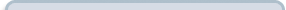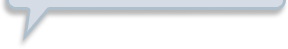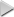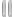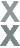# Fan Appreciation Night, Part 1

###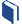Resources for this lesson: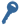Key Terms:

Theoretical probability
Independent
Dependent

> Glossary> Calculator Resources> Teacher Resources: Instructional Notes## Create and Analyze

A second option is to explore the theoretical probability of answering each of the questions correctly. When examining theoretical probabilities of multiple events, it is important to determine if the events are independent (when the outcome of one event does not influence the outcome of the other event) or dependent (when the outcome of one event impacts the outcome of the other event).  If two events are independent, the probability of both events occurring can be found by finding the product of each individual event, so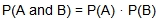. As they reflect, Khalid finds two number cubes on the teacher’s desk. He and Andrew roll the number cubes.

## Test and Confirm

Once you select a strategy, it is time to apply the strategy to determine the probability of answering 8 history questions correctly.

##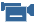Real-Life Scenarios

> Text version for animation## Apply the Model

From the theoretical results, it is clear that while Khalid may be able to answer the majority of the history questions, the chance of him answering all of the questions correctly is low.